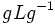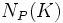# Every p-subgroup is conjugate to a p-subgroup whose normalizer in the Sylow is Sylow in its normalizer

A related survey article is: finding conjugate subgroups

## Statement

Suppose$G$ is a finite group and$P$ is a$p$-Sylow subgroup of$G$. Suppose$H$ is a subgroup contained inside$P$. Then, there exists a subgroup$K$ of$P$, such that$H$ and$K$ are conjugate subgroups inside$G$, and such that$N_P(H)$ is a Sylow subgroup of$N_G(H)$.

## Proof

Given: A finite group$G$, a$p$-Sylow subgroup$P$, a subgroup$H$ contained inside$P$.

To prove: There exists a subgroup$K$ of$P$ such that$H$ and$K$ are conjugate subgroups inside$G$, and such that ,math>N_P(H)[/itex] is$p$-Sylow inside$N_G(H)$.

Proof:

1. Let$L$ be a$p$-Sylow subgroup of$N_G(H)$. Such a$L$ exists by fact (1) in the subgroup$N_G(H)$.
2. Let$g \in G$ be such that$L \le g^{-1}Pg$. Note that such a$g$ exists by fact (2) in the group$G$.
3. Let$K = gHg^{-1}$. Then,$gLg^{-1}$ is a ,math>p[/itex]-Sylow subgroup of$N_G(K)$. By construction,$gLg^{-1} \le P$, so a$p$-Sylow subgroup of$N_G(K)$ is contained in$P$. Thus,$N_P(K)$ is$p$-Sylow in$N_G(K)$.